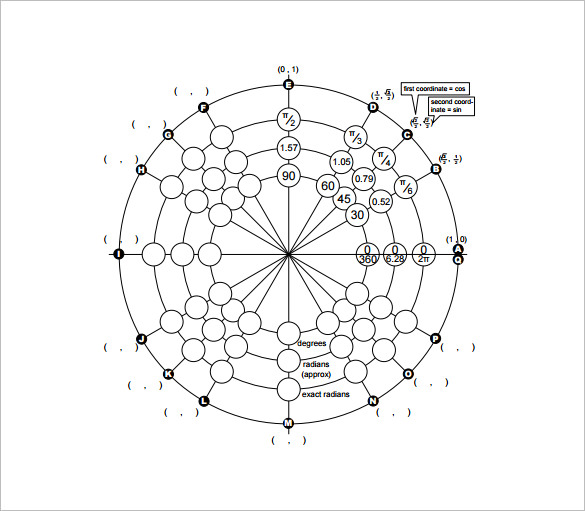# 20+ Unit Circle Chart Templates

Unit circle chart constitutes a significant teaching aide for the maths teachers in schools. Such a chart especially helps to teach the trigonometry facts & position of angles precisely. Are you a maths teacher who needs to come up with unit circle chart for your class? A good idea here would be to free download Unit Circle Charts available over the internet.

## 8 Unit Circle Chart Templates Bundle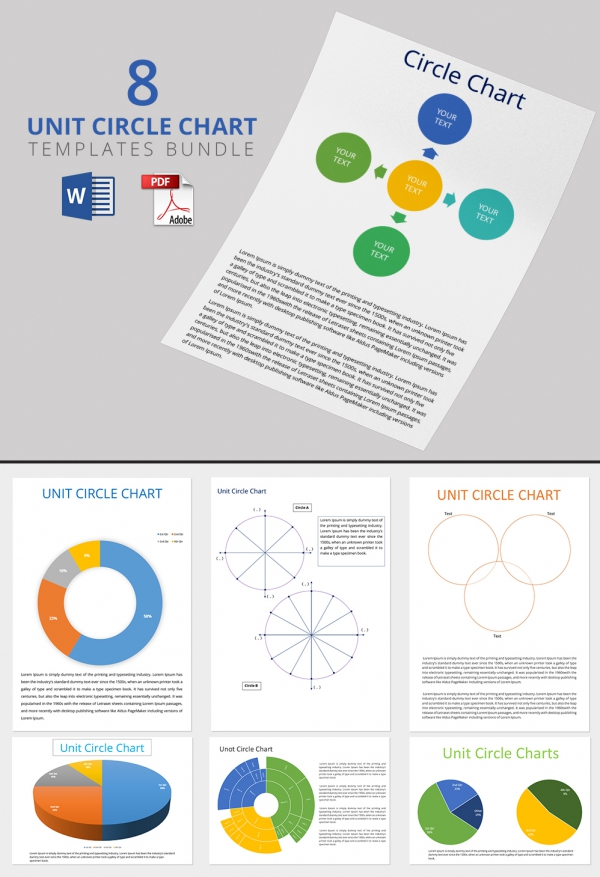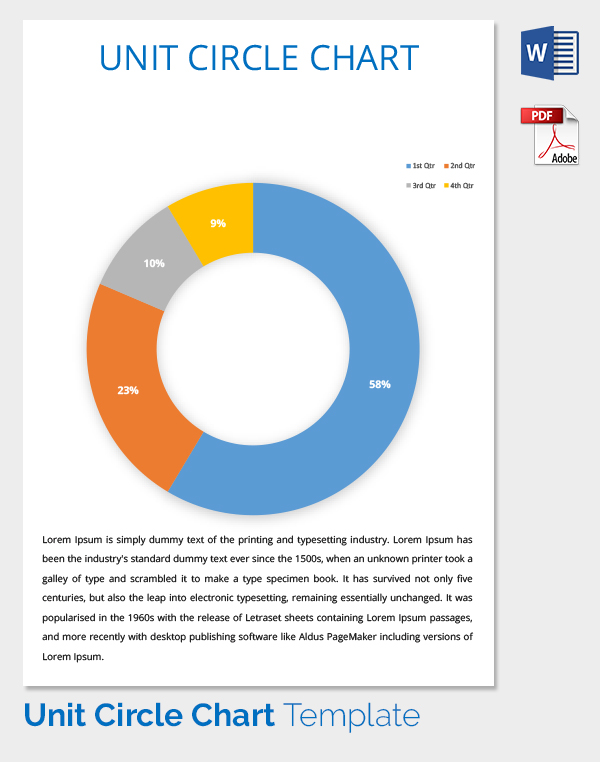## Simple Unit Circle Chart Template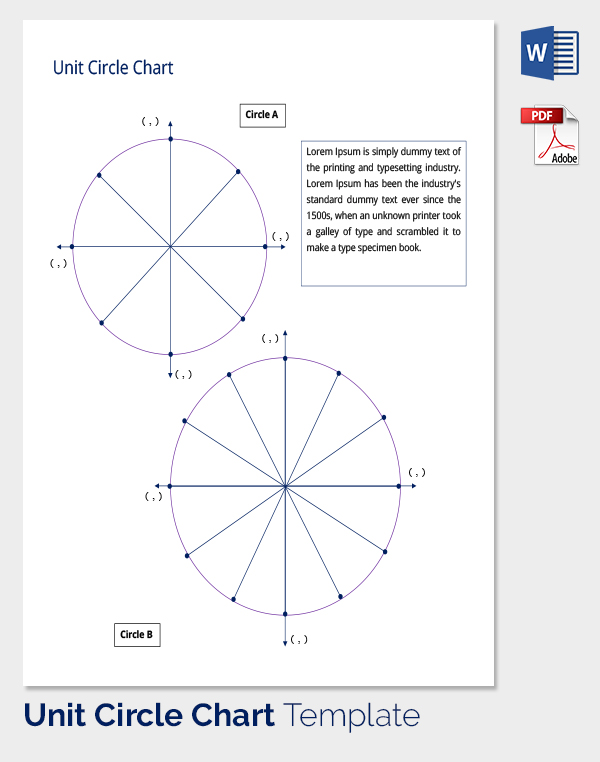## Editable Unit Circle Chart Template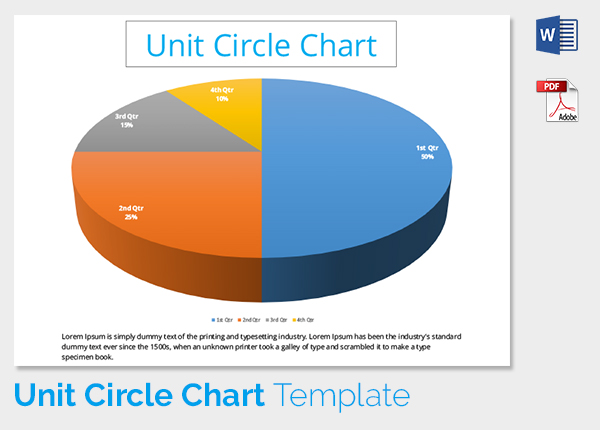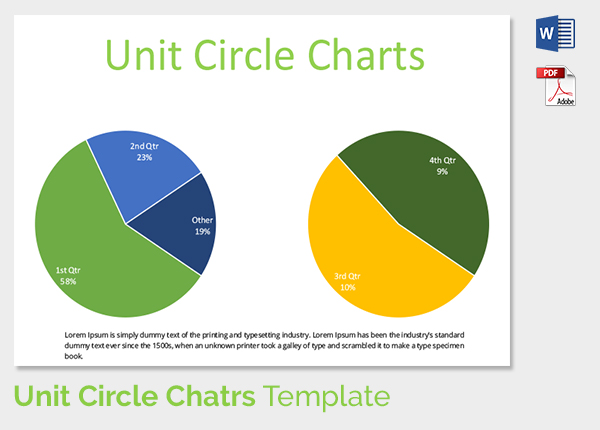## Printable Unit Circle Chart Template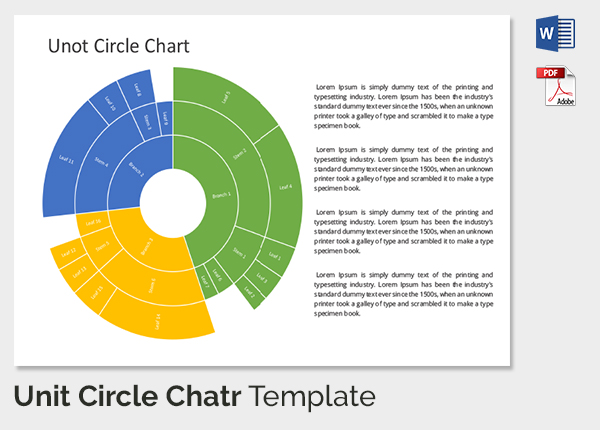## Editable Unit Circle Chart Template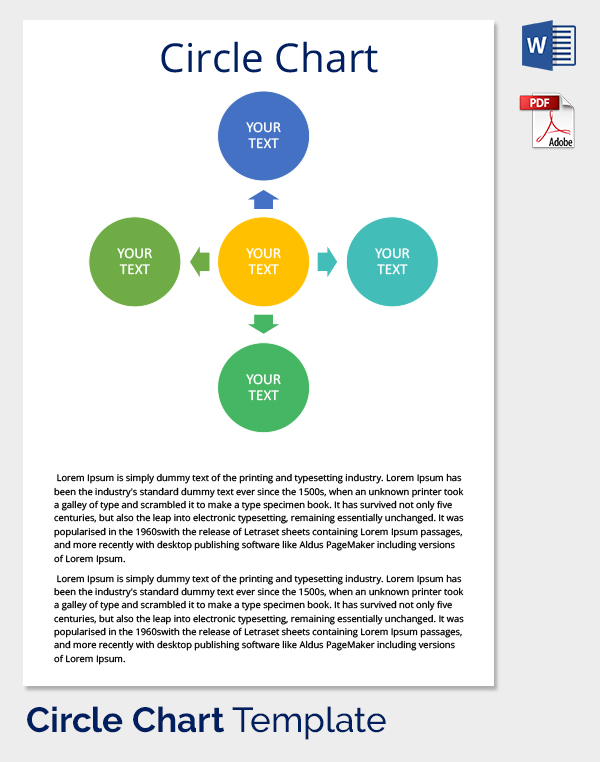## Unit Circle Chart Template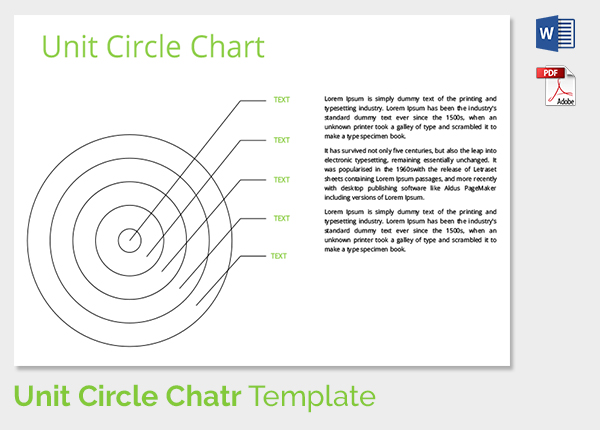## Blank Unit Circle Chart Template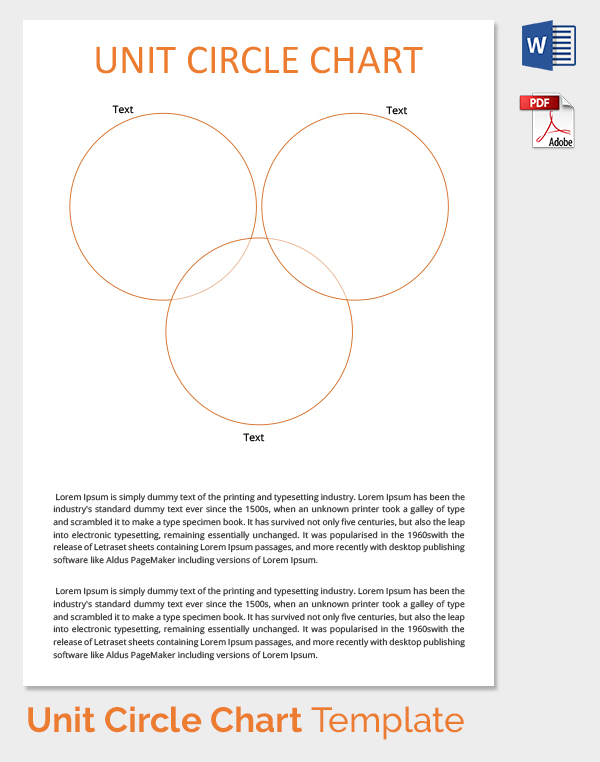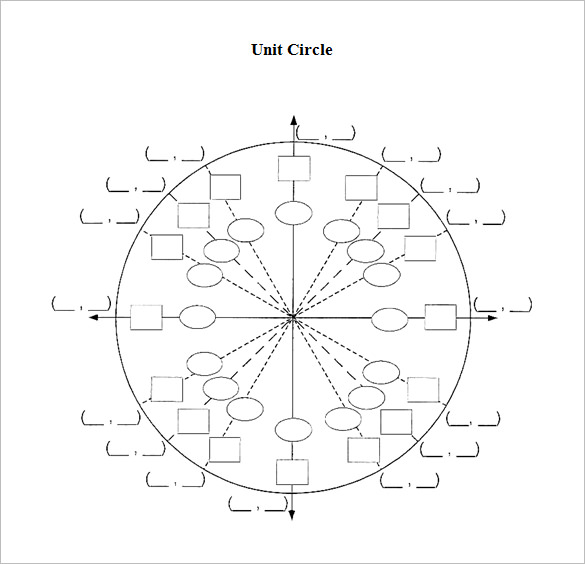You are getting an elaborate unit circle chart here which comes with a circle and all the angles drawn inside the circle. There are blank spaces to be filled with the data on each angle. northallegheny.org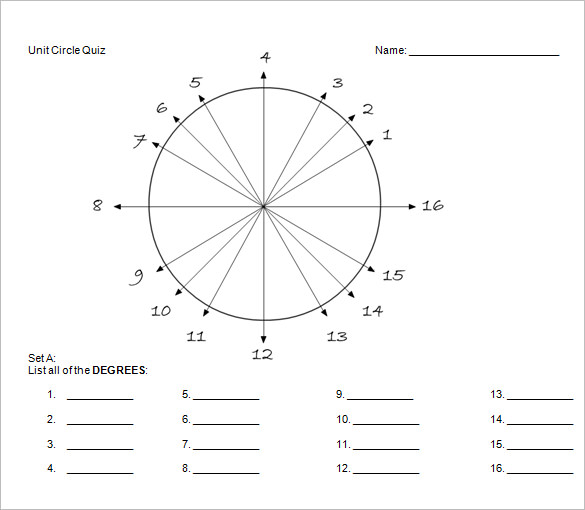designatedderiver.wikispaces.com | Unit circle chart quiz is a quiz chart that can be used to test the knowledge of the students. The sixteen angles denoted by the arrowed lines in the circle are to be found out in this chart.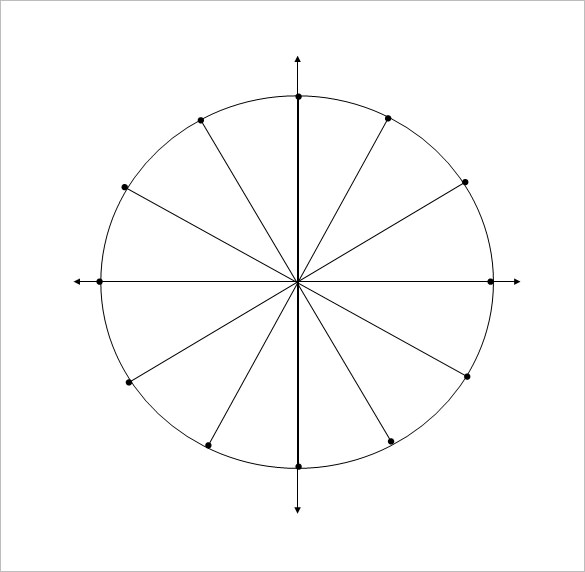This is a plain basic unit circle chart for your trigonometry class. You can paste it on the blackboard and ask the students to label the different angles drawn inside the circle. math.wsu.edu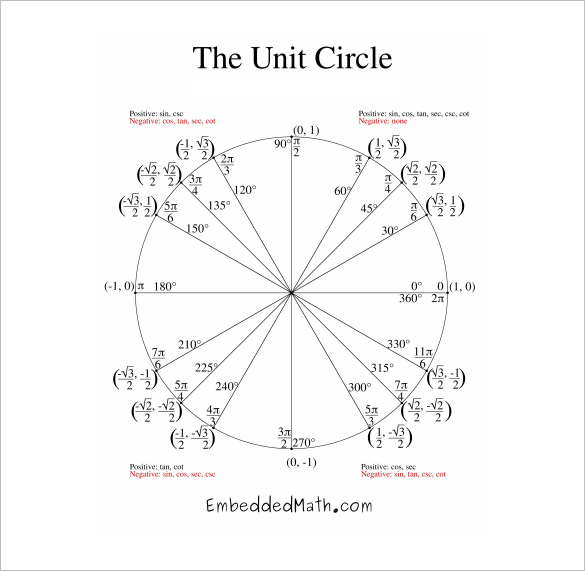collinshill.org This complete unit circle chart contains all the value of the angels. It also denotes the trigonometric values all the angles. A perfect chart to understand the basic of trigonometry and circle.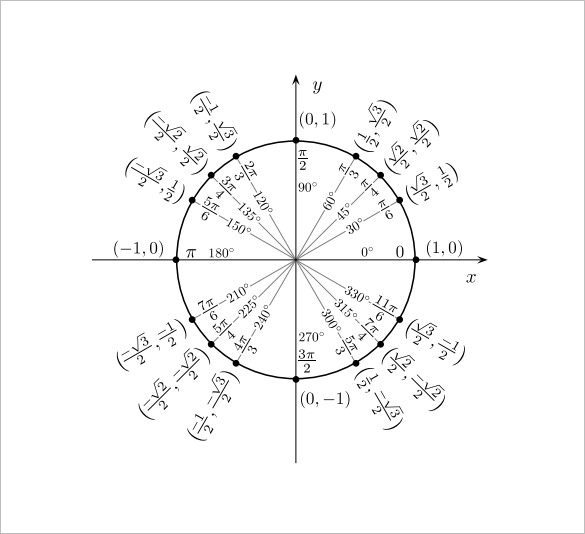evergreen.edu If you have to teach unit circle to your class, this charts with radians and degrees would be really handy for you. It’s a great time saver as you won’t have to put any extra effort on it.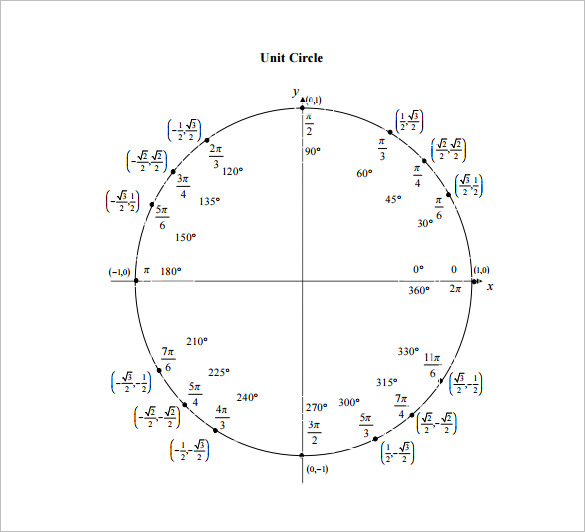tutorial.math.lamar.edu This is a basic unit circle trigonometry chart which comes with just the basic right angles and their radians. If you don’t want to get elaborate with all the angles in one single class, this template is for you.